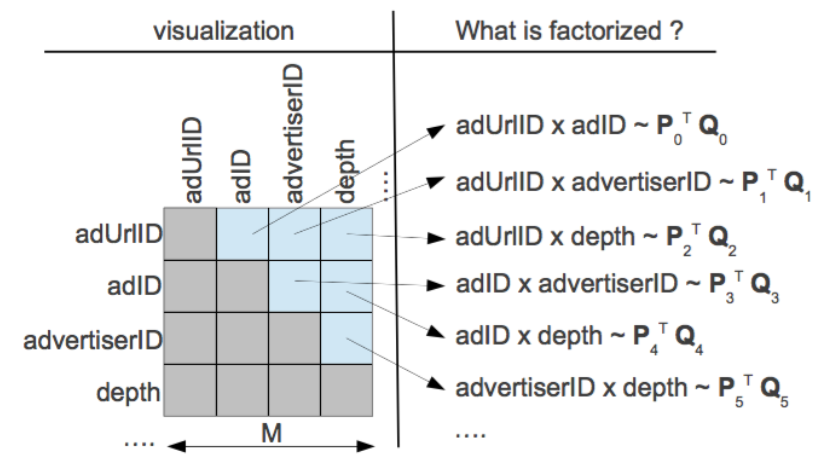## 推荐系统的简单模型

$$\hat{r}_{ui} = q_i^T p_u + \mu + b_i + b_u$$

$$A_{m \times n} = U_{m \times r}V_{r \times n}^T$$$$A_{n \times n} = Q \lambda Q^{-1}$$

## 奇异值分解

$$A_{m\times n}=U_{m\times k}\Sigma_{k\times k}V^T_{k\times n}$$

$$(AA^T)u_i = \lambda_i u_i$$

$$(A^TA)v_i = \lambda_i v_i$$

$$A=U\Sigma V^T \Rightarrow AV=U\Sigma V^TV \Rightarrow AV=U\Sigma \Rightarrow Av_i = \sigma_i u_i \Rightarrow \sigma_i = Av_i / u_i$$

$$A_{m \times n} = U_{m \times m}\Sigma_{m \times n} V^T_{n \times n} \approx U_{m \times k}\Sigma_{k \times k} V^T_{k \times n}$$$$C = \sum_{(u,i)\in{R}}(r_{u,i} - b_i - b_u - u_u*v_i^T)^2 + \lambda(\parallel {u_u} \parallel ^2 + \parallel{v_i}\parallel ^2 + b_u^2 + b_i^2)$$

$$r_{u,i} = \mu + b_i + b_u + v_i^T * (u_u + \mid{I_u}\mid^{-\frac{1}{2}}* \sum_{j\in I_u}{y_j})$$

$$\begin{equation} \begin{split} C = \sum_{(u,i)\in{R}}(a_{u,i} - u - b_u - b_i - v_i^T* (u_u + \min I_u \mid^{-\frac{1}{2}}\sum_{j \in I_u}y_j))^2 \ • \lambda(\sum_u(bi_u^2 + \parallel{u_u}\parallel^2) + \sum_i(b_i^2 + \parallel{v_i}\parallel^2 + \parallel{y_j}\parallel^2))) \end{split} \end{equation}$$## 计算相似度

### 协同过滤算法

#### 基于物品的协同过滤

（item-based collaborative filtering，ItemCF）

$$w_{ij}=\frac{|N(i)\cap N(j)|}{|N(j)|}$$

$$w_{ij}=\frac{|N(i)\cap N(j)|}{\sqrt{|N(j)||N(i)|}}$$

#### 基于用户的协同过滤

（User-based collaborative filtering，UserCF）

Jaccard相似度：

$$w_{u,v}= \frac{|N(u)\cap N(v)|}{|N(u)\cup N(v)|}$$

$$w_{u,v}= \frac{|N(u)\cap N(v)|}{\sqrt{|N(u)||N(v)|}}$$

## imdb电影评论推荐例子

header = ['user_id', 'item_id', 'rating', 'timestamp']  # 用户id，物品id，评分，评论时间

n_users = df.user_id.unique().shape  #用户数量
n_items = df.item_id.unique().shape  #物品数量
print('Number of users = ' + str(n_users) + ' | Number of movies = ' + str(n_items))
# 数据集分割——训练集：测试集 = 3:1
train_data,test_data = train_test_split(df, test_size = 0.25)

train_data_matrix = np.zeros((n_users, n_items))
test_data_matrix = np.zeros((n_users, n_items))
#使用 pandas 遍历行数据
for line in train_data.itertuples():
#训练集评分矩阵
train_data_matrix[line-1, line-1] = line
for line in test_data.itertuples():
#测试集评分矩阵
test_data_matrix[line-1, line-1] = line

user_similarity = pairwise_distances(train_data_matrix, metric = "cosine")  # 计算余弦距离
item_similarity = pairwise_distances(train_data_matrix.T, metric = "cosine")

def predict(rating, similarity, type = 'user'):
if type == 'user':
#
mean_user_rating = rating.mean(axis = 1)    #mean函数：压缩列，对各行求均值，返回 m *1 矩阵
# print(mean_user_rating)
rating_diff = (rating - mean_user_rating[:,np.newaxis])
pred = mean_user_rating[:,np.newaxis] + similarity.dot(rating_diff) / np.array([np.abs(similarity).sum(axis=1)]).T
#dot函数：矩阵相乘；np.abs()：矩阵元素的绝对值  .T:转置
# print('test',pred.min())
elif type == 'item':
pred = rating.dot(similarity) / np.array([np.abs(similarity).sum(axis=1)])
# print('test2',pred.max())
return pred
item_prediction = predict(train_data_matrix, item_similarity, type = 'item')
user_prediction = predict(train_data_matrix, user_similarity, type = 'user')
print(len(item_prediction))
print(len(item_prediction))
print(np.argsort(item_prediction))

def rmse(prediction, ground_truth):
# 计算均方误差
#nonzero(a)返回数组a中值不为零的元素的下标
#flatten()创建矩阵
prediction = prediction[ground_truth.nonzero()].flatten()
ground_truth = ground_truth[ground_truth.nonzero()].flatten()
return sqrt(mean_squared_error(prediction, ground_truth))
print('User based CF RMSE: ' + str(rmse(user_prediction, test_data_matrix)))
print('Item based CF RMSE: ' + str(rmse(item_prediction, test_data_matrix)))

SVD分解的方法，其中k是特征值个数，指定将分解为$mk$和$kn$，中间的对角矩阵有k个特征值

k: int, optional,Number of singular values and vectors to compute. Must be 1 <= k < min(A.shape)

u, s, vt = svds(train_data_matrix, k=500)
s_diag_matrix = np.diag(s)
X_pred = np.dot(np.dot(u, s_diag_matrix), vt)

print('User-based CF MSE: ' + str(rmse(X_pred, test_data_matrix)))